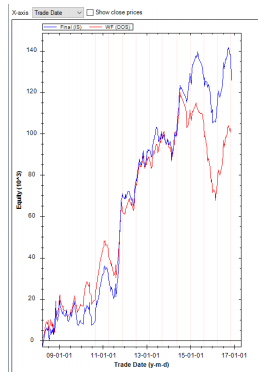## Folder: “Graphs” – Equity curves

The equity curves show this:- Final (IS) curve in blue: see “Optimization Summary (IS)” run=Final. This equity curve is built based on the                     parameter settings in run=Final over the entire IS period.

- WF (OOS) in red: this equity curve is built adding up all OOS runs (see Folder: “Walk-Forward Summary                     (OOS).

Options for the equity curves:

- X-axis: On this pull-down menu either “Trade Date” (as per above example) or Trade Number can be selected.

- “Show close prices” can be ticked. This will introduce a third equity curve with the close prices for the instrument             being traded. In the above example this is un-ticked.